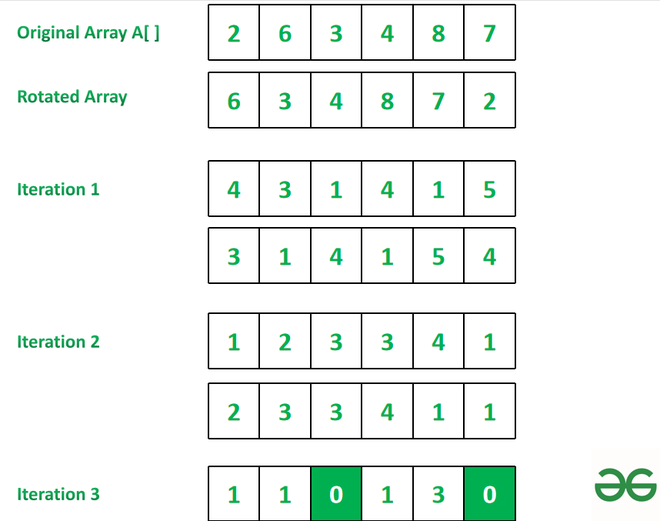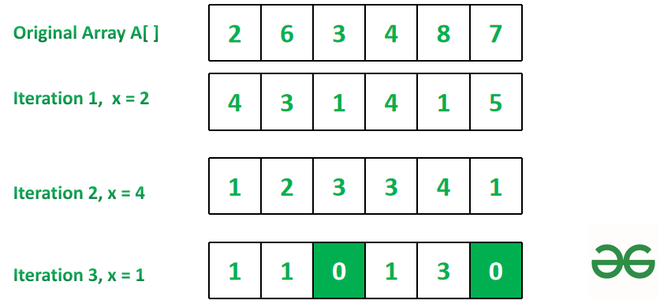# Count of iterations to make minimum as 0 by rotating Array followed by reducing it from original Array

• Difficulty Level : Medium
• Last Updated : 16 Feb, 2022

Given an array arr[]. The task is to find the number of iterations required to make the minimum element in the array as 0. In one iteration, left rotate the array by one and subtract the corresponding element of the original array and rotated array.

Examples:

Input: arr[] = { 2, 6, 3, 4, 8, 7 }
Output: 3
Explanation: Refer to the image below for explanation.Input: arr[] = { 4, 10, 12, 3, 9, 7 }
Output: 5

Naive Approach: The easiest way to solve this problem is by using the Greedy Approach.

• Simply pop the first element of the array and append it to the end and then perform the subtraction on the corresponding element.
• Similarly, perform the same operation on the resultant array till we get minimum element in an array as zero, and return the count of iteration.

Below is the implementation of the above approach

## C++

 `// C++ program for above approach``#include ``using` `namespace` `std;` `// Function to find no of iterations.``int` `minZero(vector<``int``>& A, ``int` `n)``{` `    ``// Initialize count c = 0.``    ``int` `c = 0;` `    ``// if zero is already present``    ``// in array return c.``    ``if` `(*min_element(A.begin(), A.end()) == 0)``        ``return` `c;` `    ``// Iterate till minimum``    ``// in array becomes zero.``    ``while` `(*min_element(A.begin(), A.end()) != 0) {` `        ``// Copy array element to A1``        ``vector<``int``> A1 = A;` `        ``// Pop first element and``        ``// append it to last``        ``int` `x = A;``        ``A.erase(A.begin());``        ``A.push_back(x);` `        ``// Perform subtraction``        ``for` `(``int` `i = 0; i < n; i++)``            ``A[i] = ``abs``(A[i] - A1[i]);` `        ``// Increment count by 1``        ``c += 1;``    ``}` `    ``// Return value of count c``    ``return` `c;``}` `// Driver Code``int` `main()``{``  ` `    ``// Original array``    ``vector<``int``> arr = { 2, 6, 3, 4, 8, 7 };` `    ``// Calling method minZero``    ``int` `x = minZero(arr, arr.size());` `    ``// Print the result``    ``cout << (x);` `    ``return` `0;``}` `    ``// This code is contributed by rakeshsahni`

## Java

 `// Java program for above approach``import` `java.util.*;` `class` `GFG{` `  ``// Function to find no of iterations.``  ``static` `int` `minZero(Vector A, ``int` `n)``  ``{` `    ``// Initialize count c = 0.``    ``int` `c = ``0``;` `    ``// if zero is already present``    ``// in array return c.``    ``if` `(Collections.min(A) == ``0``)``      ``return` `c;` `    ``// Iterate till minimum``    ``// in array becomes zero.``    ``while` `(Collections.min(A) != ``0``) {` `      ``// Copy array element to A1``      ``Vector A1 = (Vector) A.clone();` `      ``// Pop first element and``      ``// append it to last``      ``int` `x = A.get(``0``);``      ``A.remove(``0``);``      ``A.add(x);` `      ``// Perform subtraction``      ``for` `(``int` `i = ``0``; i < n; i++)``        ``A.set(i, Math.abs(A.get(i) - A1.get(i)));` `      ``// Increment count by 1``      ``c += ``1``;``    ``}` `    ``// Return value of count c``    ``return` `c;``  ``}` `  ``// Driver Code``  ``public` `static` `void` `main(String[] args)``  ``{` `    ``// Original array``    ``Integer []arr = { ``2``, ``6``, ``3``, ``4``, ``8``, ``7` `};``    ``Vector v = ``new` `Vector();``    ``Collections.addAll(v, arr);``    ` `    ``// Calling method minZero``    ``int` `x = minZero(v, arr.length);` `    ``// Print the result``    ``System.out.print(x);` `  ``}``}` `// This code is contributed by 29AjayKumar`

## Python3

 `# Python program for above approach` `# Function to find no of iterations.``def` `minZero(A, n):` `    ``# Initialize count c = 0.``    ``c ``=` `0` `    ``# if zero is already present``    ``# in array return c.``    ``if` `min``(A) ``=``=` `0``:``        ``return` `c` `    ``# Iterate till minimum``    ``# in array becomes zero.``    ``while` `min``(A) !``=` `0``:` `        ``# Copy array element to A1``        ``A1 ``=` `A[:]` `        ``# Pop first element and``        ``# append it to last``        ``x ``=` `A.pop(``0``)``        ``A.append(x)` `        ``# Perform subtraction``        ``for` `i ``in` `range``(n):``            ``A[i] ``=` `abs``(A[i]``-``A1[i])` `        ``# Increment count by 1``        ``c ``+``=` `1` `    ``# Return value of count c``    ``return` `c` `# Driver Code` `# Original array``arr ``=` `[``2``, ``6``, ``3``, ``4``, ``8``, ``7``]` `# Calling method minZero``x ``=` `minZero(arr, ``len``(arr))` `# Print the result``print``(x)`

## C#

 `// C# program for above approach``using` `System;``using` `System.Linq;``class` `GFG{` `  ``// Function to find no of iterations``  ``static` `int` `minZero(``int` `[]A, ``int` `n)``  ``{` `    ``// Initialize count c = 0``    ``int` `c = 0;` `    ``// If 0 already in array return c` `    ``if` `(A.Min()== 0)``      ``return` `c;` `    ``// Iterate till we get zero in array``    ``while` `(A.Min() != 0) {` `      ``// Assign first element in x``      ``int` `x = (``int``)A;` `      ``// Loop to subtract consecutive element``      ``for` `(``int` `i = 0; i < (n - 1); i++) {``        ``A[i] = Math.Abs((``int``)A[i] - (``int``)A[i + 1]);``      ``}``      ``A[n - 1] = Math.Abs((``int``)A[n - 1] - x);` `      ``// Increment count c``      ``c += 1;``    ``}` `    ``// Return c``    ``return` `c;``  ``}` `  ``// Driver Code``  ``public` `static` `void` `Main()``  ``{` `    ``// Original array``    ``int` `[]arr = { 2, 6, 3, 4, 8, 7 };` `    ``// Length of array``    ``int` `N = arr.Length;` `    ``// calling function``    ``int` `x = minZero(arr, N);` `    ``// print the result``    ``Console.Write(x);``  ``}``}` `// This code is contributed by avijitmondal1998`

## Javascript

 ``
Output
`3`

Time Complexity:  O(N)
Auxiliary Space:  O(N)

Efficient Approach: A space-optimized approach is a logic and implementation-based. Follow the steps below to solve the given problem.

• Store the first element of array in variable x.
• Now find absolute difference between consecutive elements.
• Replace result from index 0.
• Subtract last element from variable x and store it.
• count the iteration and repeat the steps.
• Return count as the final answer.Below is the implementation of the above approach

## C++

 `// C++ program for above approach``#include ``using` `namespace` `std;` `// Function to find no of iterations``int` `minZero(``int` `A[], ``int` `n)``{` `    ``// Initialize count c = 0``    ``int` `c = 0;` `    ``// If 0 already in array return c` `    ``if` `(*min_element(A + 0, A + n - 1) == 0)``        ``return` `c;` `    ``// Iterate till we get zero in array``    ``while` `(*min_element(A + 0, A + n - 1) != 0) {` `        ``// Assign first element in x``        ``int` `x = A;` `        ``// Loop to subtract consecutive element``        ``for` `(``int` `i = 0; i < (n - 1); i++) {``            ``A[i] = ``abs``(A[i] - A[i + 1]);``        ``}``        ``A[n - 1] = ``abs``(A[n - 1] - x);` `        ``// Increment count c``        ``c += 1;``    ``}` `    ``// Return c``    ``return` `c;``}` `// Driver Code``int` `main()``{``  ` `    ``// Original array``    ``int` `arr[] = { 2, 6, 3, 4, 8, 7 };` `    ``// Length of array``    ``int` `N = ``sizeof``(arr) / ``sizeof``(arr);` `    ``// calling function``    ``int` `x = minZero(arr, N);` `    ``// print the result``    ``cout << (x);``}` `// This code is contributed by ukasp.`

## Java

 `// Java program for above approach``import` `java.util.*;``class` `GFG{` `// Function to find no of iterations``static` `int` `minZero(``int` `A[], ``int` `n)``{` `    ``// Initialize count c = 0``    ``int` `c = ``0``;` `    ``// If 0 already in array return c` `    ``if` `(Arrays.stream(A).min().getAsInt()== ``0``)``        ``return` `c;` `    ``// Iterate till we get zero in array``    ``while` `(Arrays.stream(A).min().getAsInt() != ``0``) {` `        ``// Assign first element in x``        ``int` `x = A[``0``];` `        ``// Loop to subtract consecutive element``        ``for` `(``int` `i = ``0``; i < (n - ``1``); i++) {``            ``A[i] = Math.abs(A[i] - A[i + ``1``]);``        ``}``        ``A[n - ``1``] = Math.abs(A[n - ``1``] - x);` `        ``// Increment count c``        ``c += ``1``;``    ``}` `    ``// Return c``    ``return` `c;``}` `// Driver Code``public` `static` `void` `main(String[] args)``{``  ` `    ``// Original array``    ``int` `arr[] = { ``2``, ``6``, ``3``, ``4``, ``8``, ``7` `};` `    ``// Length of array``    ``int` `N = arr.length;` `    ``// calling function``    ``int` `x = minZero(arr, N);` `    ``// print the result``    ``System.out.print(x);``}` `}` `// This code is contributed by 29AjayKumar`

## Python3

 `# Python program for above approach` `# Function to find no of iterations``def` `minZero(A, n):` `    ``# Initialize count c = 0``    ``c ``=` `0` `    ``# If 0 already in array return c``    ``if` `min``(A) ``=``=` `0``:``        ``return` `c` `    ``# Iterate till we get zero in array``    ``while` `min``(A) !``=` `0``:` `        ``# Assign first element in x``        ``x ``=` `A[``0``]` `        ``# Loop to subtract consecutive element``        ``for` `i ``in` `range``(n``-``1``):``            ``A[i] ``=` `abs``(A[i]``-``A[i ``+` `1``])``        ``A[n``-``1``] ``=` `abs``(A[n``-``1``]``-``x)` `        ``# Increment count c``        ``c ``+``=` `1` `    ``# Return c``    ``return` `c` `# Driver Code` `# Original array``arr ``=` `[``2``, ``6``, ``3``, ``4``, ``8``, ``7``]` `# Length of array``N ``=` `len``(arr)` `# calling function``x ``=` `minZero(arr, N)` `# print the result``print``(x)`

## C#

 `// C# program for above approach``using` `System;``using` `System.Linq;``class` `GFG{` `// Function to find no of iterations``static` `int` `minZero(``int` `[]A, ``int` `n)``{` `    ``// Initialize count c = 0``    ``int` `c = 0;` `    ``// If 0 already in array return c` `    ``if` `(A.Min()== 0)``        ``return` `c;` `    ``// Iterate till we get zero in array``    ``while` `(A.Min() != 0) {` `        ``// Assign first element in x``        ``int` `x = (``int``)A;` `        ``// Loop to subtract consecutive element``        ``for` `(``int` `i = 0; i < (n - 1); i++) {``            ``A[i] = Math.Abs((``int``)A[i] - (``int``)A[i + 1]);``        ``}``        ``A[n - 1] = Math.Abs((``int``)A[n - 1] - x);` `        ``// Increment count c``        ``c += 1;``    ``}` `    ``// Return c``    ``return` `c;``}` `// Driver Code``public` `static` `void` `Main()``{``  ` `    ``// Original array``    ``int` `[]arr = { 2, 6, 3, 4, 8, 7 };` `    ``// Length of array``    ``int` `N = arr.Length;` `    ``// calling function``    ``int` `x = minZero(arr, N);` `    ``// print the result``    ``Console.Write(x);``}``}` `// This code is contributed by Samim Hossain Mondal.`

## Javascript

 ``
Output
`3`

Time Complexity: O(N)
Auxiliary Space: O(1)

My Personal Notes arrow_drop_up